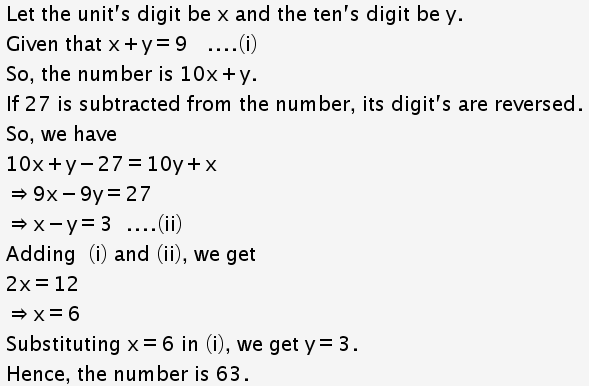# A number consist of two digits whose sum is 9. If 27 is subtracted from the number its digits are reversed. Find the number?

A number consist of two digits whose sum is 9. If 27 is subtracted from the number its digits are reversed. Find the number?